# Determine Unconfined Compressive Strength of Cohesive SoilReading time: 1 minute

The unconfined compressive strength (qu) is the load per unit area at which the cylindrical specimen of a cohesive soil falls in compression.

qu = P/A

Where P= axial load at failure, A= corrected area =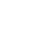, where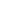is the initial area of the specimen,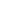= axial strain = change in length/original length. The undrained shear strength (s) of the soil is equal to the one half of the unconfined compressive strength,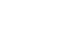## Determine Unconfined Compressive Strength of Cohesive Soil

### Equipment for the Test

1. Unconfined compression apparatus, proving ring type
2. Proving ring, capacity 1 kN, accuracy 1N.
3. Dial gauge, accuracy 0.01 mm.
4. Weighing balance
5. Oven
6. Stop watch
7. Sampling tube
8. Split mould, 38mm diameter, 76mm long.
9. Sample extractor
10. Knife.
11. Vernier calipers
12. Large mould.

### Procedure of Unconfined Compressive Strength Test

1. Place the sampling soil specimen at the desired water content and density in the large mould.
2. Push the sampling tube into the large mould and remove the sampling tube filled with the soil. For undisturbed samples, push the sampling tube into the clay sample.
3. Saturate the soil sample in the sampling tube by a suitable method.
4. Coat the split mould lightly with a thin layer of grease. Weigh the mould.
5. Extrude the sample out of the sampling tube into the split mould, using the sample extractor and the knife.
6. Trim the two ends of the specimen in the split mould. Weigh the mould with the specimen.
7. Remove the specimen from the split mould by splitting the mould into two parts.
8. Measure the length and diameter of the specimen with vernier calipers.
9. Place the specimen on the bottom plate of the compression machine. Adjust the upper plate to make contact with the specimen.
10. Adjust the dial gauge and the proving ring gauge to zero.
11. Apply the compression load to cause an axial strain at the rate of ½ to 2% per minute.
12. Record the dial gauge reading, and the proving ring reading every thirty seconds up to a strain of 6%. The reading may be taken after every 60 seconds for a strain between 6%, 12% and every 2minutes or so beyond 12%.
13. Continue the test until failure surfaces have clearly developed or until an axial strain of 20% is reached.
14. Measure the angle between the failure surface and the horizontal, if possible.
15. Take the sample from the failure zone of the specimen for the water content determination.Fig: Unconfined Compression Testing Machine (Spring Type)Fig: Unconfined Compression Testing Machine (Proving Ring Type)

### Data Sheet for Unconfined Compression Test

Initial length of the specimen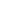= Initial diameter of the specimen,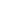= Initial area of the specimen,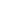= Initial volume of the specimen,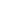= Mass of empty split mould = Mass of split mould + specimen = Mass of the specimen M = Bulk density,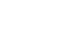= Water content = Dry density= Specific gravity of solids, G= Void ratio,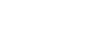Degree of saturation,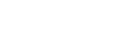Plot a curve between the compressive stress as ordinate, and axial strain as abscissa.Fig: Mohr’s Circle for Unconfined Compression Test

Results of the Test: From the plot, unconfined compressive strength,= Shear strength,=